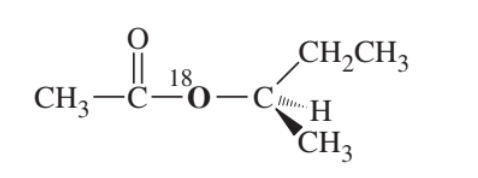×
Get Full Access to Organic Chemistry - 8 Edition - Chapter 21 - Problem 16p
Get Full Access to Organic Chemistry - 8 Edition - Chapter 21 - Problem 16p

×

# Suppose we have some optically pure (R)-2-butyl acetateISBN: 9780321768414 33

## Solution for problem 16P Chapter 21

Organic Chemistry | 8th Edition

• Textbook Solutions
• 2901 Step-by-step solutions solved by professors and subject experts
• Get 24/7 help from StudySoup virtual teaching assistantsOrganic Chemistry | 8th Edition

4 5 1 371 Reviews
26
2
Problem 16P

Suppose we have some optically pure-butyl acetate that has been "labeled" with the heavy $${ }^{18} O$$ isotope at one oxygen atom as shown.(a) Draw a mechanism for the hydrolysis of this compound under basic conditions. Predict which of the products will contain the $${ }^{18} O$$ label. Also predict whether the butan- 2 -ol product will be pure, pure, or racemized.

(b) Repeat part (a) for the acid-catalyzed hydrolysis of this compound.

(c) Explain how you would prove experimentally where the $${ }^{18} O$$ label appears in the products. ($${ }^{18} O$$ is not radioactive.Equation transcription:Text transcription:

^{18} O

Step-by-Step Solution:
Step 1 of 3

Chemistry Lecture 7 Chapter 15: Chemical Equilibrium Dynamics of Chemical Reactions and Microscopic Reversibility  Reactions can be reversible and dynamic  when rate of forward and reverse reaction are equal  reaction arrows in each direction can denote this Equilibrium  does not mean same amount of reactant and product  concentrations do not need to be equal  can go in favor of products or reactants (can be determined by which you have relatively more of)  an expression  equilibrium constant =K eq (make sure its a capital K)  equilibrium constant only changes when temperature changes  Rate constant does not have to be equal for forward reaction and reverse reaction  when equilibrium is reached, the concentration of the products and reactants no longer change Rules for calculating  coefficients in front of the reactant turn into the exponents when plugging into equation  products are always divided by reactants  any products or reactants that are solid or liquid should not be included in the equation  only include reactants and products in the gaseous or aqueous state The I.C.E. Table  I­ inital concentration  C­ change in concentration  E­ equilibrium concentration  The initial concentration of produc

Step 2 of 3

Step 3 of 3

##### Edition: 8
The full step-by-step solution to problem: 16P from chapter: 21 was answered by , our top Chemistry solution expert on 05/06/17, 06:41PM. Since the solution to 16P from 21 chapter was answered, more than 374 students have viewed the full step-by-step answer. The answer to “?Suppose we have some optically pure -butyl acetate that has been "labeled" with the heavy $${ }^{18} O$$ isotope at one oxygen atom as shown. (a) Draw a mechanism for the hydrolysis of this compound under basic conditions. Predict which of the products will contain the $${ }^{18} O$$ label. Also predict whether the butan- 2 -ol product will be pure , pure , or racemized.(b) Repeat part (a) for the acid-catalyzed hydrolysis of this compound.(c) Explain how you would prove experimentally where the $${ }^{18} O$$ label appears in the products. ($${ }^{18} O$$ is not radioactive. Equation transcription:Text transcription:^{18} O” is broken down into a number of easy to follow steps, and 102 words. Organic Chemistry was written by and is associated to the ISBN: 9780321768414. This full solution covers the following key subjects: pure, predict, products, label, hydrolysis. This expansive textbook survival guide covers 25 chapters, and 1336 solutions. This textbook survival guide was created for the textbook: Organic Chemistry, edition: 8.